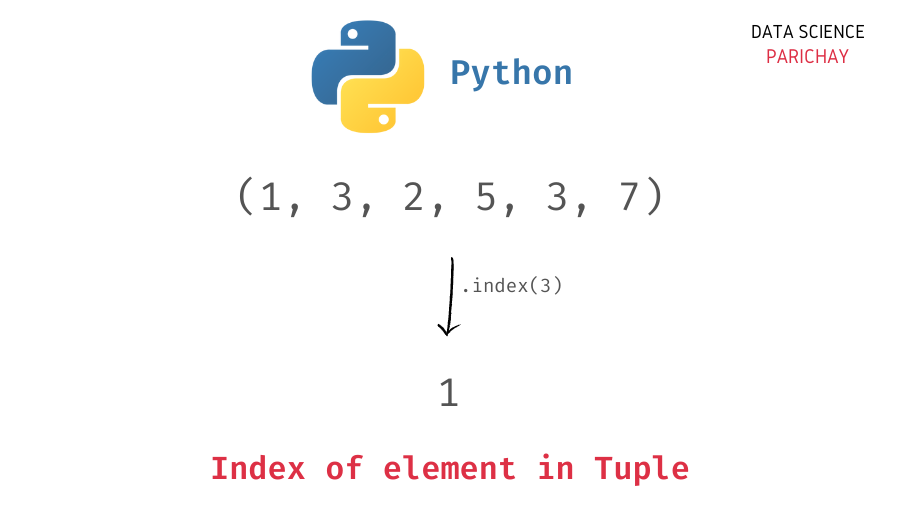# Find Index of an Element in a Tuple in Python

In this tutorial, we will look at how to find the index of an element in a tuple in Python with the help of some examples.

You can use the Python tuple `index()` function to find the index of an element in a tuple. The following is the syntax.

```# find index of element e in tuple t
t.index(e)```

It returns the index of the first occurrence of the value inside the tuple. If the value is not present in the tuple, it gives an error.

Let’s look at some examples.

Let’s find the index of the element `3` inside the tuple `(1, 3, 2, 5, 3, 7)`.

```# create a tuple
t = (1, 3, 2, 5, 3, 7)
# find index of 3
print(t.index(3))```

Output:

`1`

We get the index of `3` as `1`. Note that the element `3` is present twice inside the tuple, at indexes `1` and `4` respectively but the index function only returned the index of its first occurrence, that is, `1`.

If you want all the indexes of occurrences of a value, you can iterate over the values in a loop. For example, let’s find all the indexes of `3` in the above tuple.

📚 Data Science Programs By Skill Level

Introductory

Intermediate ⭐⭐⭐

🔎 Find Data Science Programs 👨‍💻 111,889 already enrolled

Disclaimer: Data Science Parichay is reader supported. When you purchase a course through a link on this site, we may earn a small commission at no additional cost to you. Earned commissions help support this website and its team of writers.

```# create a tuple
t = (1, 3, 2, 5, 3, 7)
# find all indexes of 3
result = []
for i in range(len(t)):
if t[i] == 3:
result.append(i)
print(result)```

Output:

`[1, 4]`

We get both its indexes. Here we iterate over each value in the tuple and compare it with `3`, if it’s equal, we add its index to our result list.

You can use a list comprehension to reduce the above code to a single line.

```# create a tuple
t = (1, 3, 2, 5, 3, 7)
# find all indexes of 3
result = [i for i in range(len(t)) if t[i]==3]
print(result)```

Output:

`[1, 4]`

We get the same result as above.

If the element is not present inside the tuple, the `index()` function will give an error. For example, let’s try to find the index of `4`, an element that is not present in the above tuple.

```# create a tuple
t = (1, 3, 2, 5, 3, 7)
# find index of 4
print(t.index(4))```

Output:

```---------------------------------------------------------------------------
ValueError                                Traceback (most recent call last)
Input In , in <module>
2 t = (1, 3, 2, 5, 3, 7)
3 # find index of 4
----> 4 print(t.index(4))

ValueError: tuple.index(x): x not in tuple```

We get a `ValueError` indicating that the element for which we are trying to find the index is not present in the tuple.

You might also be interested in –

•